One end of a string 4.32 m long is moved up and down with simple harmonic motion at a frequency of 75 Hz . The waves reach the other end of

Question

One end of a string 4.32 m long is moved up and down with simple harmonic motion at a frequency of 75 Hz . The waves reach the other end of the string in 0.5 s. Find the wavelength of the waves on the string. Answer in units of cm.

in progress 0
6 months 2021-07-31T17:11:30+00:00 2 Answers 5 views 0

1. To solve this problem, we will apply the concepts related to the kinematic equations of linear motion, which define speed as the distance traveled per unit of time. Subsequently, the wavelength is defined as the speed of a body at the rate of change of its frequency. Our values are given as,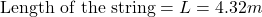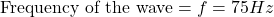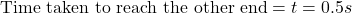Velocity of the wave,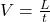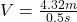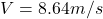Wavelength of the wave,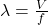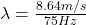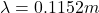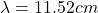Therefore the wavelength of the waves on the string is 11.53 cm

11.52cm

Explanation:

The velocity, v, of a wave undergoing a simple harmonic motion is related to the wavelength, λ, and frequency, f, of the wave as follows;

v =  f x λ         —————–(i)

But;

The velocity is also given as the ratio of the length, l, of the body producing the wave to the time taken, t, to undergo the motion. i.e

v =————–(ii)

Now, substitute the value of v in equation (ii) into equation (i) as follows;= f x λ           —————-(iii)

From the question,

l = 4.32m

t = 0.5s

f = 75Hz

Substitute these values into equation (iii) as follows;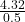= 75 x λ

Make λ subject of the formula;

λ =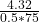λ = 0.1152m

Convert the value to cm by multiplying by 100

λ = 0.1152 x 100cm = 11.52cm

Therefore, the wavelength of the waves on the string is 11.52cm